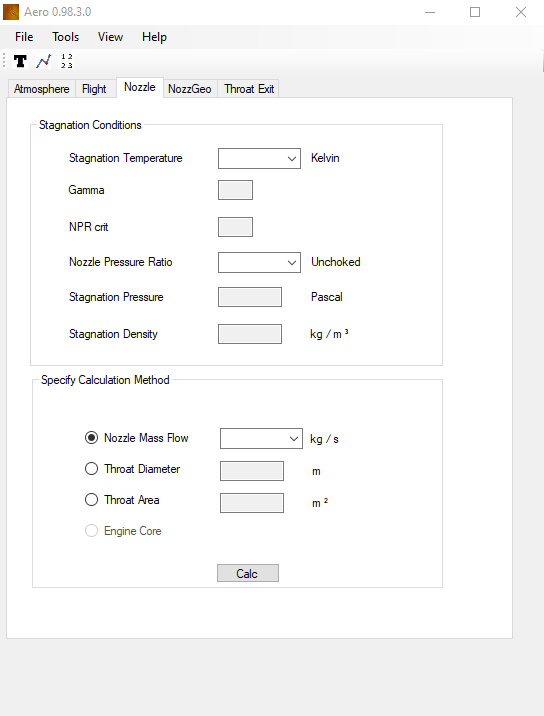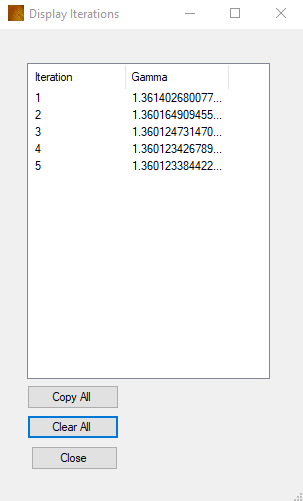CaidedTab 4 - Nozzle

Enter a resevoir stagnation temperature into the first text entry box, in Kelvin.

The ratio of specific heats (gamma) is automatically calculated and displayed.
The critical Nozzle Pressure Ratio is also calculated and displayed.

Enter a Nozzle Pressure Ratio (NPR) into fourth text entry box. This is the ratio of the stagnation pressure to the ambient static pressure. The static pressure at Altitude is used for this.

If the NPR is greater than the critical pressure ratio then the label to the side of the NPR text box will change from unchoked to choked.
This is a condition when further downstream pressure reductions do not give a larger nozzle mass flows as the flow becomes sonic at the minimum aerodynamic area.
Greater mass flows can be achieved after choking by increasing the upstream pressure and correspondingly the density increases.

The stagnation pressure and density are automatically calculated.As the static temperature at the throat is not known until the calculation has been performed Gamma is not known upfront .
As gamma is a function of temperature ideally this ought to be modified for the throat static temperature.

Therefore Gamma is iteratively solved at the Throat and used for the calculations, by calculating an initial Throat Static temperature and then modifying Gamma. This new Gamma is then used in the nozzle calculation.

Go to the Tools Option menu, and check the Debug checkbox. when the Calc button is clicked on the nozzle tab the following dialogue box will display showing the values for Gamma at each iteration.
Only five are performed as it converges rapidly.
Specify Calculation Method

Four possiblites are offered to the user to calculate the nozzle parameters.

1 - Nozzle Mass Flow
If you know the mass flow rate (kg/s) then enter it here and the program will return the Throat Area and Throat Diameter (assumes round nozzle)

2 - Throat Diameter
If you know the Throat diameter the nozzle mass flow and Throat area are calculated

3 - Throat Area
If you know the Throat Area the Thorat diameter and Nozzle mass flow are calculated

4 - Engine Core
This is greyed out until the Engine Tab is selected. With the engine Tab selected an Engine Core mass flow is entered into the Nozzle Mass Flow entry. The other parameters are then calculated

Press the calc button to perform the calculation - this is not automatically updated as per other tabs.